>Next: (b) LexA and SOS Up: 3. Results Previous: 3. Results

## (a) Ribosomes and Ribosome Binding Sites

We aligned the sequences of 149 E. coli and coliphage ribosome binding sites by their initiation codons because the process of initiation requires that the fmet-tRNA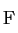bind there. Since ribosomes search mRNA, we used the composition of the transcript library (Stormo et al., 1982a) to calculate Hg: A=29526, C=25853, G=27800, T=28951 for which Hg=1.99817 bits/base. The frequencies of bases at each position of the sites were used to find the information content, Rsequence(L), as a function of position (equations 2, 3 and a.8). Fig. 2 shows that the largest peak is for the initiation codon. The second largest peak represents the "Shine and Dalgarno" sequence (Shine and Dalgarno, 1974). There are at least five other distinct peaks.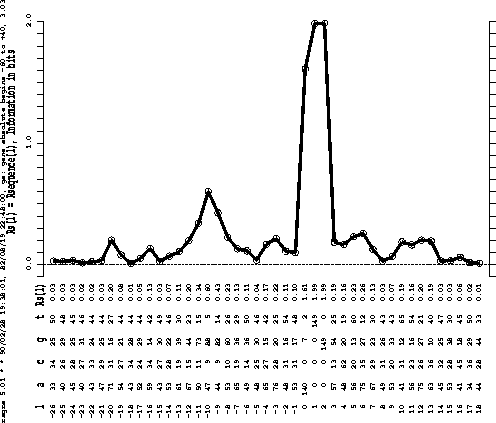Position 0 is the first base of the initiation codon.

Rsequence, the total information content of the site, is found by adding together the individual information contents from each position (equation 6). Previous statistical analyses showed a range of -21 to +13 (zero is the first base of the initiation codon), which corresponds well to the regions of RNA protected by ribosomes from ribonucleases (Gold et al., 1981). This range was extended by 5 bases on both sides. For this range, we calculate an Rsequence of 11.0 bits per site. Alignment by the Shine and Dalgarno sequence gives less than 8.3 bits (data not shown), which suggests that this is not a good alignment.

A good estimate for the size of the E. coli genome is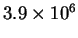basepairs (Bachmann and Low, 1980). In determining Rfrequency, we assume that almost all of the genome is transcribed into messages and that for the most part only one strand is transcribed. The number of potential ribosome binding sites is therefore. Based on the coding capacity versus DNA insert size of 24 plasmids selected at random from the Clark-Carbon bank (P. Bloch, personal communication; F.C. Neidhardt et al., 1983), and a genome size ofbp, we estimate the number of proteins encoded by E. coli, and therefore the number of ribosome binding sites, to be 2574. Equation (9) therefore gives an Rfrequency of 10.6 bits per site. The data for all analyses are gathered in Table 1.

Table 1: Information content of several molecular binding sites.
 Organism Recognizer Type n Range Rs S.D.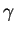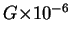Rf Rs/Rf Rs-Rf E. coli Ribosome A 149 -26 to 18 11.0 0.1 2574 3.9 10.6 1.0 0.4 E. coli LexA E 14 -9 to 10 21.1 0.6 22 7.8 18.4 1.1 2.7 E. coli TrpR E 6 -18 to 19 23.4 1.9 6 7.8 20.3 1.1 3.0 E. coli LacI O 2 -21 to 21 19.2 2.8 2 7.8 21.9 0.9 -2.6 E. coli ArgR E 16 -9 to 10 16.4 0.5 22 7.8 18.4 0.9 -2.0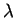cI/Cro O 12 -9 to 9 17.1 0.7 12 7.8 19.3 0.9 -2.2 T7 RNA Pol A 17 -29 to 12 35.4 0.7 83 7.8 16.5 2.1 18.9 T7 Symmetry E 34 -6 to 7 16.4 0.2 34 7.8 17.8 0.9 -1.4
Type of site: A = asymmetric, E = symmetric without a central base (Even), 0 = symmetric with a central base (Odd). n is the number of sequenced sites (for symmetric sites, both strands are counted). The range is the region over which Rsequence is calculated. Rs stands for Rsequence. S.D. is the standard deviation of Rsequence owing to small sample size; the variance of information content for individual sites will be presented elsewhere.is the number of distinct binding sites in the genome. For symmetrical sites, there are two possible ways to bind, sois twice the number of conventional sites. G is the number of potential binding sites on the genome. Rf stands for Rfrequency. Calculations were carried out to five decimal places and then rounded.Next: (b) LexA and SOS Up: 3. Results Previous: 3. Results
Tom Schneider
2002-10-16